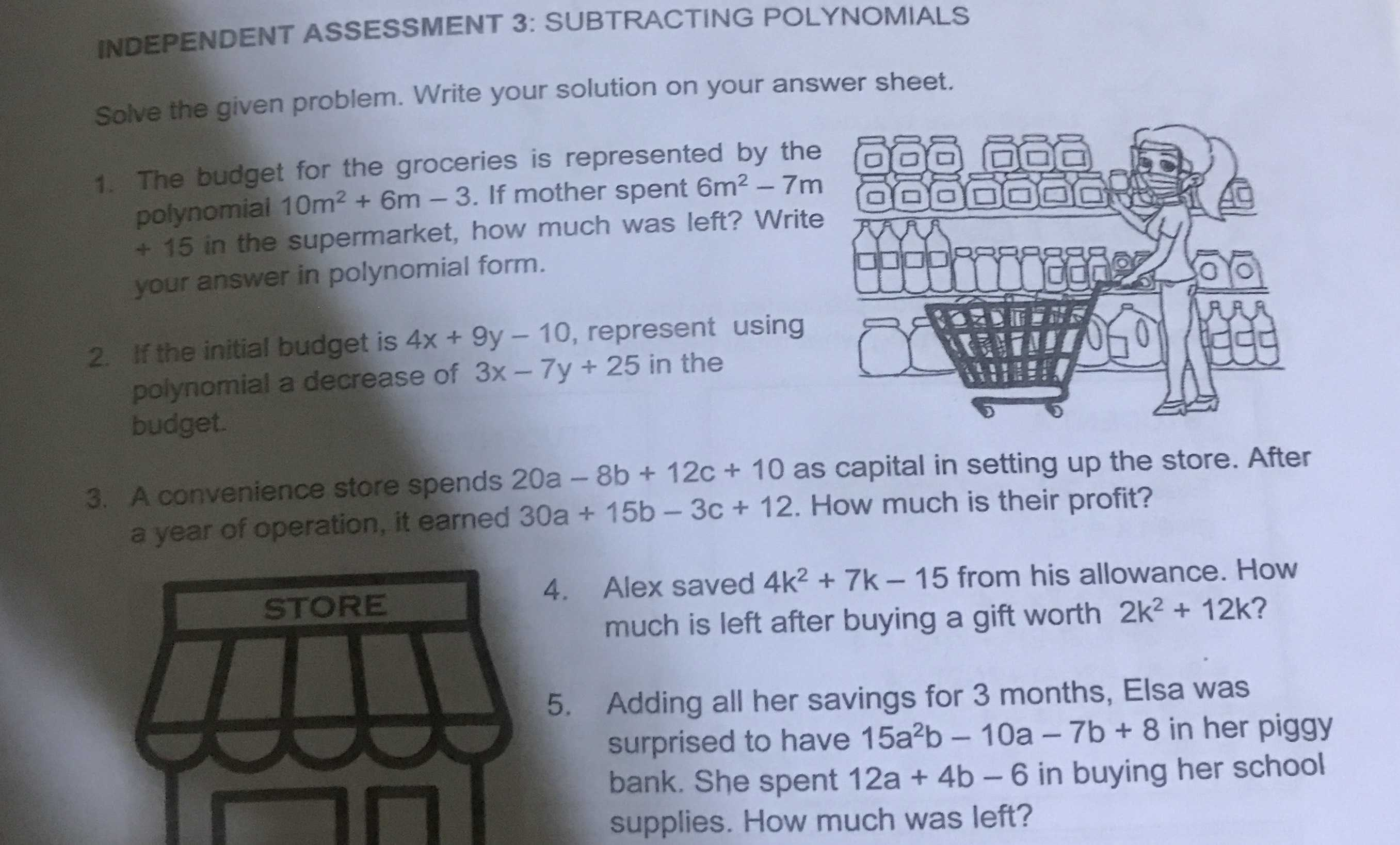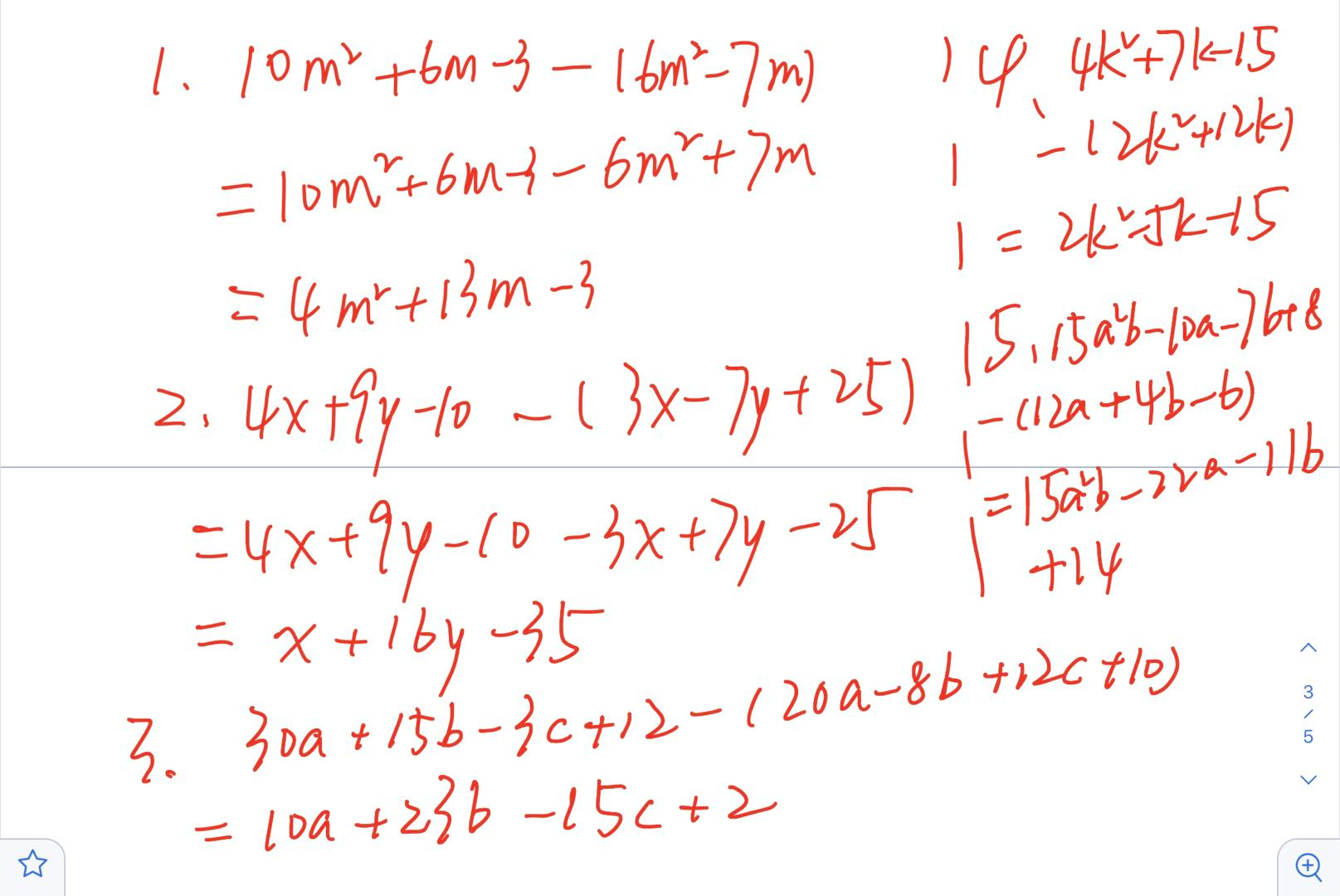### ¿Todavía tienes preguntas de matemáticas?

Pregunte a nuestros tutores expertos
Algebra
Pregunta1. The budget for the groceries is represented by the polynomial $$10 m ^ { 2 } + 6 m - 3$$ . If mother spent $$6 m ^ { 2 } - 7 m$$ $$+ 15$$ in the supermarket, how much was left? Write your answer in polynomial form.

2. If the initial budget is $$4 x + 9 y - 10$$ , represent using polynomial a decrease of $$3 x - 7 y + 25$$ in the budget.

3. A convenience store spends $$20 a - 8 b + 12 c + 10$$ as capital in setting up the store. After a year of operation, it earned $$30 a + 15 b - 3 c + 12$$ . How much is their profit?